# The statistical foundations of machine learningDeveloping machine learning algorithms is easier than ever. There are several high-level libraries like TensorFlow, PyTorch, or scikit-learn to build upon. Thanks to the fantastic effort of many talented developers, these are easy to use and require only a superficial familiarity with the underlying algorithms. However, this comes at the cost of deep understanding. Without proper theoretical foundations, one quickly gets overwhelmed with complex technical details.

My aim is to demonstrate how seemingly obscure and ad-hoc methods in machine learning can be explained with elementary and natural ideas when we have the proper perspective. Our mathematical tool for that will be probability theory and statistics, which lies at the foundation of predictive models. Using the theory of probability is not just an academic exercise. It can provide profound insight into how machine learning works, giving you the tools to improve on state-of-the-art.

Before we begin our journey in the theoretical foundations of machine learning, let's look at a toy problem!

## Fitting models

Suppose that we have two numerical quantities, say $\textstyle x$ and $\textstyle y$, that are in relation to each other. For instance, $\textstyle x$ is the square footage of a real estate, and $\textstyle y$ is its price in the market for a given city. Our goal is to predict $\textstyle y$ from $\textstyle x$. In mathematical terms, we can say that we are looking for a function $f(x)$ such that

$\widehat{y} = f(x)$

is as close as possible to the ground truth. The simplest assumption is that the function is linear, that is, $f(x) = ax + b$ for some $\textstyle a$ and $\textstyle b$ constants. This model surprisingly fits well with many real-life problems. Moreover, it is a fundamental building block of more complex models such as neural networks.

Our example dataset looks like the following.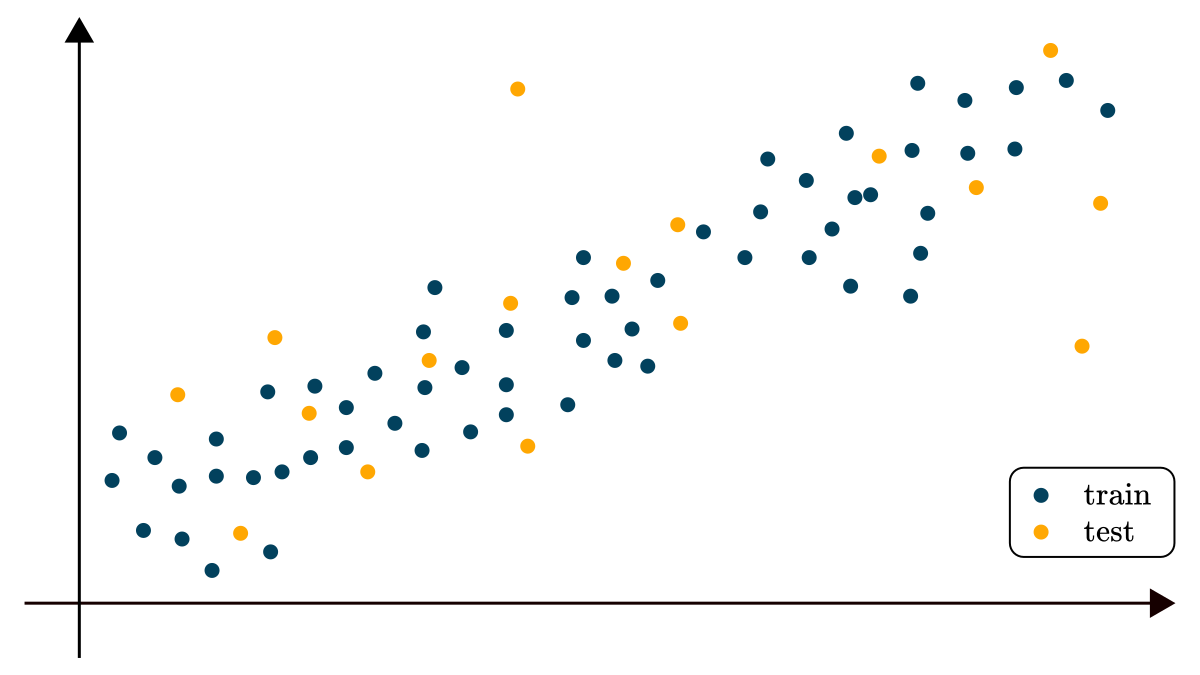Suppose that our dataset consists of data points and observations

${(x_i, y_i)}_{i=1}^{n}$

Since data is noisy, it is impossible to fit a linear model to our data for which

$f(x_i) = y_i, \quad i = 1, 2, \dots, n$

holds. Instead, we measure how well a particular model fits the data and find the best fit possible according to that measurement.

Most frequently, this is done by calculating the prediction error for each point, then averaging the pointwise error. The lower the error, the better our model is. The simplest way to measure this is to take the square of the differences between prediction and ground truth. This is called the mean square error, and it is defined by

\begin{align*} \text{MSE}(a, b) &= \frac{1}{n} \sum_{i=1}^{n} \big( y_i - f(x_i) \big)^2 \\ &= \frac{1}{n} \sum_{i=1}^{n} (y_i - ax_i - b)^2. \end{align*}

The best fit is the one where the mean square error assumes its minimum, or in mathematical terms,

$a, b = \text{argmin}_{a, b} \text{MSE}(a, b).$

For our concrete dataset, this looks like the following.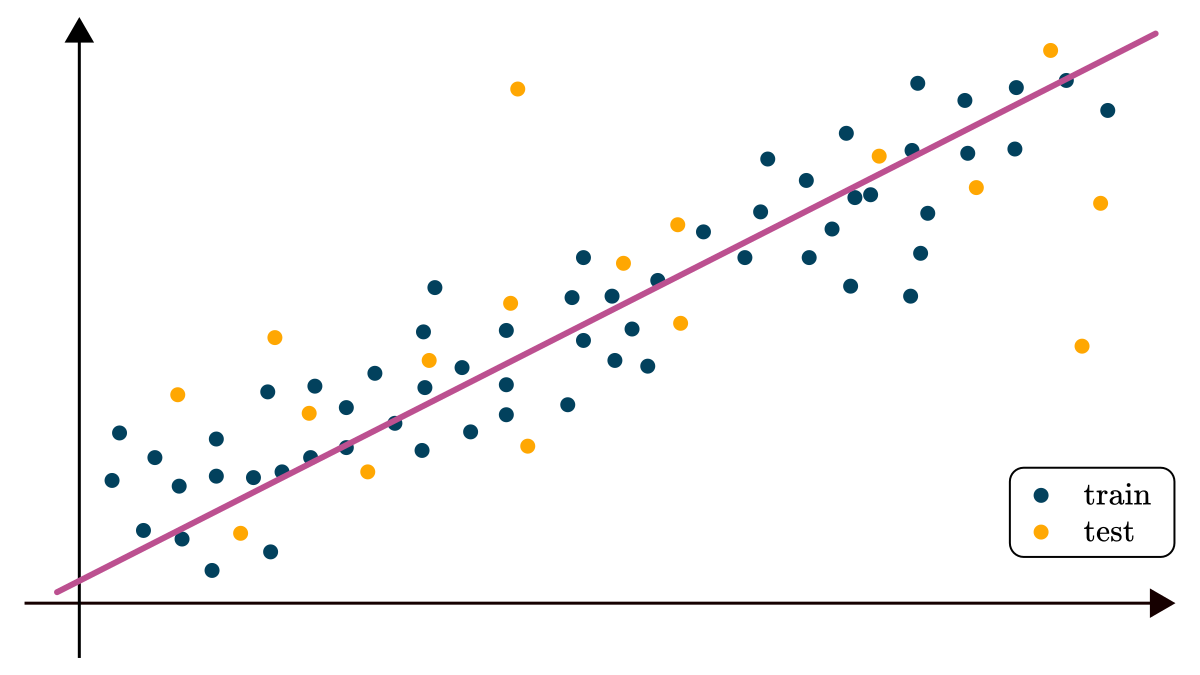How to minimize this is not particularly important for our purposes. What matters to us is how the model can be interpreted and what kind of information it conveys. By simply looking at it, one can tell that there are many missing things. Sure, the data follows a linear trend, but can this even be described with our model? A function is deterministic: you plug in $\textstyle x$, you get the prediction $f(x)$. However, the data seems to show some noise that our linear model does not capture adequately.

Where else might we look? One obvious answer would be to look for a more complex function, say a higher degree polynomial. In mathematical terms, we are looking for a function defined by

$f(x) = \sum_{i=0}^{d}a_i x^i,$

which can hopefully describe the data more precisely. Unfortunately, this is not the case.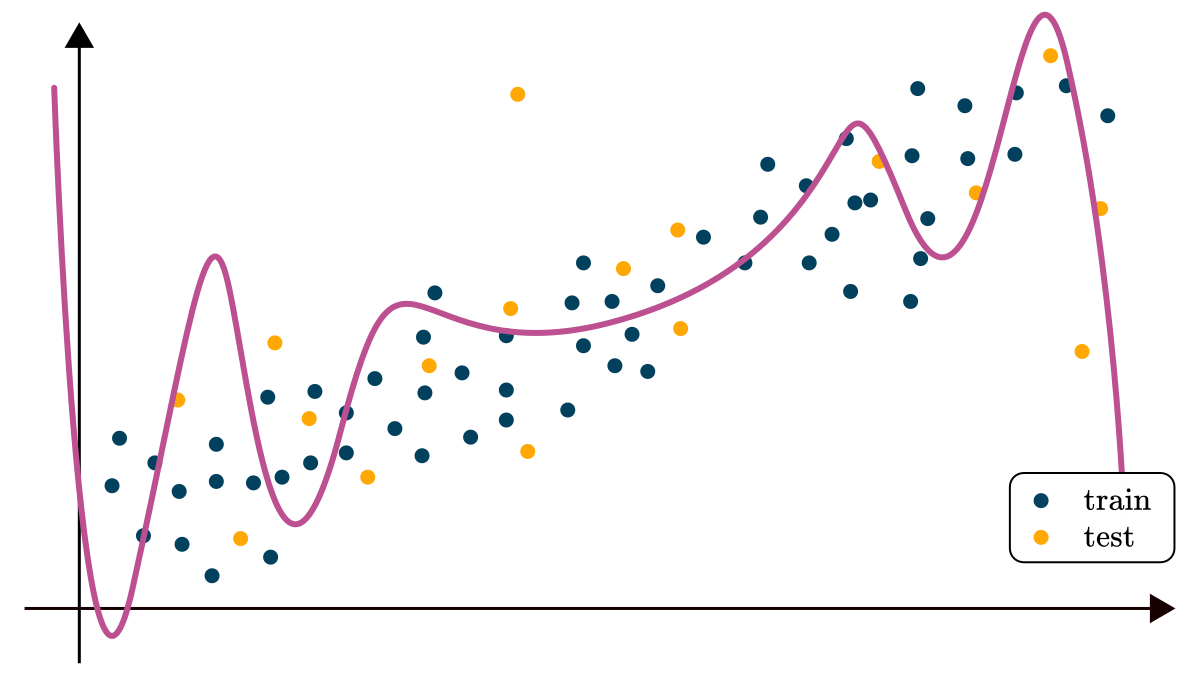When we try to brute force it and excessively increase the expressive power of our model, we see that in a sense, things are even worse: our concerns are not addressed, and other issues are introduced, like the wild behavior of the model outside our domain. We have to look in different directions for the solution. Instead of looking for a single value as a prediction, we should aim for a model explaining the probability of specific outcomes! This way, we can make a more informed decision, such as calculating risks.

## The language of probability theory

Suppose that I have a coin with 1/2 probability of coming up heads and 1/2 probability of coming up tails upon a coin toss. If I toss the coin 100 times, how many heads will I get? It might be tempting to answer 50 quickly, but the reality is not so simple. See, you can get any number of heads from 0 to 100, but these are not equally probable. In one experiment, you might get 34, in the next, 52, etc. The probability of heads being 1/2 means that if you keep tossing the coins infinitely, the proportion of heads vs. all tosses will get closer and closer to 1/2.

Let's stick to the example of tossing a coin $\textstyle n$ times! Suppose that $\textstyle X$ denotes the number of heads. $\textstyle X$ is a random variable for which we do not know the exact value, only the probability of assuming a given value. The probability that $\textstyle X$ is $\textstyle k$ is denoted by $P(X = k)$, always a number between 0 and 1. Moreover, since we know that we can get either 0, 1, 2, and so on up until n heads, these probabilities sum to 1, that is,

$\sum_{k=1}^{n} P(X = k) = 1.$

We can describe every experiment with an $\textstyle n$-long sequence of $\textstyle H$-s and $\textstyle T$-s. To calculate the exact probability for a given $\textstyle k$, we must count how many ways we can have $\textstyle k$ heads among $\textstyle n$ tosses. This is mathematically equivalent to selecting a subset of $\textstyle k$ elements from a set of $\textstyle n$ elements, which can be done in

$\binom{n}{k} = \frac{n!}{k! (n - k)!}$

ways. Moreover, each configuration has $(1/2)^n$ probability. (For example, consider $n = 3$. The configuration $HTH$ has probability 1/8, since each particular toss has 1/2 probability in itself and the tosses are independent.) So putting this together, we have

$P(X = k) = \binom{n}{k} \frac{1}{2^n}$

Together, these numbers are referred to as the probability distribution of the experiment. (Which is tossing $\textstyle n$ coins and observing the number of heads among them.)

To summarize, a probability distribution encompasses all information about the outcome of the experiment. Our model in the previous section only gives us a single number. However, to make a fully informed decision, we should be looking for a probability distribution instead.

### The general Bernoulli and Binomial distributions

Our two examples above can be generalized. First, suppose that we toss an unfair coin, that is, a coin where heads and tails don't have equal probability. Say, the probability of heads is $\textstyle p$ and denote the number of heads with $\textstyle X$ as before. (Which can be zero or one.)

$P(X = 1) = p, \quad P(X = 0) = 1 - p$

is called the Bernoulli distribution with parameter $\textstyle p$, or $Bernoulli(p)$ in short. Following this line of thinking, if we toss up this unfair coin $\textstyle n$ times, we have

$P(X = k) = \binom{n}{k} p^k (1 - p)^{n - k}$

which is called the binomial distribution with parameters $\textstyle n$ and $\textstyle p$, or $b(n, p)$ in short. Note that the Bernoulli distribution is just the binomial distribution with $n = 1$.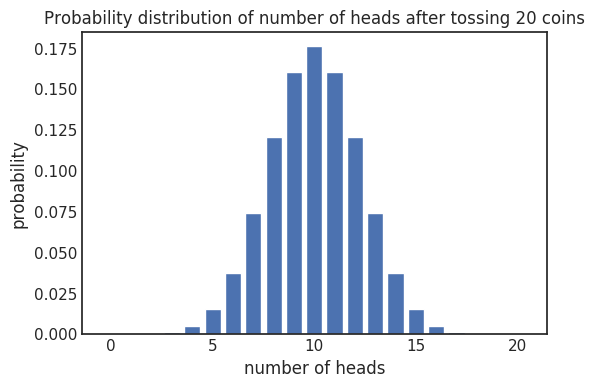### Continuous probability distributions

In the previous coin-tossing examples, probability distributions were fully described by the numbers $P(X = k)$ for all feasible $\textstyle k$. This particular random variable can only assume integers as its values, of which there are countably many. Such random variables are called discrete. But what about the previous case, where we estimated real estate prices? In general, random variables can also assume all real numbers as their values, for instance. In this case, they are called continuous. Say $\textstyle X$ can be any random number between zero and one with equal probability. What is its probability distribution? Intuitively, we can see that

$P(X = x) = 0$

for any fixed $\textstyle x$ in $[0, 1]$. So, how would we describe this distribution? We use the so-called probability density function (PDF in short), which describes the probability that $\textstyle X$ falls into a certain range, say $a \leq x \leq b$. The probability itself can be calculated by measuring the area under the curve of the PDF between $\textstyle a$ and $\textstyle b$. In the case of selecting a random number between zero and one, we have

$P_X(x) = \begin{cases} 1 & \text{if } 0 \leq x \leq 1, \\ 0 & \text{otherwise} \end{cases}$

for the density function. Note that the total area under the density function graph is always $\textstyle 1$ because it expresses the probability of all outcomes.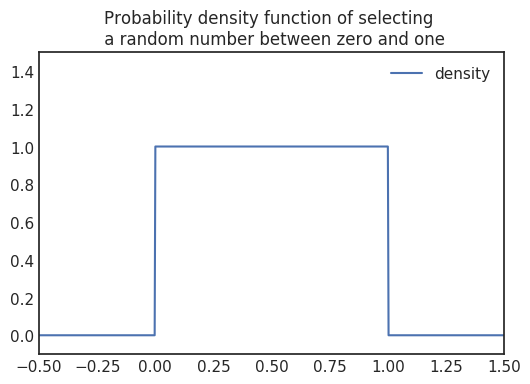### The normal distribution

A continuous distribution of great importance is the so-called normal distribution. (Or Gaussian in another name.) You have encountered this at some point, even though you may not have known that it was a normal distribution. We say that $\textstyle X$ is normally distributed with mean $\textstyle \mu$ and variance $\textstyle \sigma^2$ variance, or $\mathcal{N}(\mu, \sigma^2)$ in short, if its density function is

$P_X(x) = \frac{1}{\sigma \sqrt{2 \pi}} e^{- \frac{(x - \mu)^2}{2\sigma^2}}.$

This comes up very frequently in real life. For instance, the height of people tends to show this distribution. We will encounter this many times later in our journey. The mean describes the center of the bell curve.

Notation-wise, the PDF of $\mathcal{N}(\mu, \sigma^2)$ is frequently denoted as $\mathcal{N}(x | \mu, \sigma^2)$. We will use this later.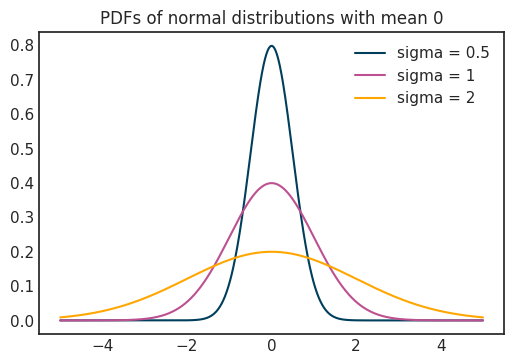### Conditional probability

Let's consider a different example now. Instead of tossing coins, we will roll a single six-sided dice. If we denote the outcome of the throw with $\textstyle X$, it is reasonable to assume that

$P(X = 1) = \dots = P(X = 6) = \frac{1}{6}$

Suppose that you want to make a bet. Your friend throws the dice, and if the outcome is less or equal to 3, you win. Otherwise, you lose the same amount. It is easy to see that by default, your probability of winning is 1/2. However, your friend tells you after throwing the dice that the outcome is an even number. What about your chances of winning now? Intuitively, you have lower chances now because you can only win with a 2 and lose if it is 4 or 6. Thus, additional information on the experiment has changed the underlying probability distribution.

This concept can be formalized mathematically as the conditional probability. Let's suppose you have two events, $\textstyle A$ and $\textstyle B$. In our concrete example, $\textstyle A$ is that the outcome of the throw is less or equal than 3, while $\textstyle B$ is that the outcome of the throw is an even number. The probability of $\textstyle A$ given that $\textstyle B$ has happened is called the conditional probability of $\textstyle A$ given $\textstyle B$. It is denoted with $P(A | B)$ and can be calculated as

$P(A | B) = \frac{P(A \text{ and } B)}{P(B)}$

In our case, $P(A \text{ and } B) = 1/6$, while $P(B) = 1/2$, so our chance of winning is $P(A | B) = 1/3$.

### The statistical foundations of machine learning

We need to take another conceptual jump to see how conditional probability fits into our machine learning perspective. Let's revisit our coin-tossing example! However, this time, the coin is not fair, so the probability of heads is not 1/2. Let's assume that

$P(H) = p, \quad P(T) = 1 - P(H) = 1 - p$

for some $\textstyle p$ in $[0, 1]$. The catch is, we don't know its exact value. We have to guess from the data. In other words, we want to estimate its probability distribution. $\textstyle p$ is a parameter in our coin toss experiment. (Just to be clear, we have two distributions here: one describes the outcome of a coin toss, while the second one describes our belief about the probability of heads for our given coin.)

Suppose that we have the particular coin in our hands and we toss it up in the air ten times, obtaining the result

$\text{HHTHTHHHHT}$

that is, three tails and seven heads. In the language of probability, let $\textstyle E$ describe the event "seven heads out of ten". So, what we really want, is

$P(p | E).$

This is called the posterior since it describes our belief about the coin after observing some data. This is a continuous probability distribution since $\textstyle p$ can assume any value between zero and one. How would we calculate this? A fundamental property of conditional probability comes to the rescue here. If $\textstyle A$ and $\textstyle B$ are general events, then

\begin{align*} P(A | B) &= \frac{P(A \text{ and } B)}{P(B)} \\ &= \frac{P(A \text{ and } B) P(A)}{P(B) P(A)} \\ &= \frac{P(A \text{ and } B)}{P(A)} \frac{P(A)}{P(B)} \\ &= \frac{P(B | A) P(A)}{P(B)} \end{align*}

In other words, the probability of $\textstyle A$ conditioned on $\textstyle B$ can be expressed with the probability of $\textstyle B$ conditioned on $\textstyle A$. This is called the Bayes theorem, which holds true for probability density functions as well. How does this help us? Now we have

$P(p | E) = \frac{P(E | p) P(p)}{P(E)}$

which is great for us! There are three components in play here.

i) $P(E | p)$, which is called the likelihood. It is easy to calculate, and we did so in the previous section. In our current case with seven heads, we have

$P(E | p) \propto p^7 (1 - p)^3$

which means they are proportional to each other, that is, equal up until a multiplicative constant. This constant doesn't matter for us for reasons to be explained later.

ii) $P(p)$, which is called prior, because it expresses our knowledge about the coin before we have observed any data. It is reasonable to assume that every parameter is equally probable, so

$P(p) = \begin{cases} 1 & \text{if } 0 \leq p \leq 1 \\ 0 & \text{otherwise} \end{cases}$

which is already familiar to us.

iii) $P(E)$, which does not need to be calculated since the area under the function $P(p | E)$ is always one. (In most cases, calculating $P(E)$ is computationally intractable.) Because of this exact reason, we do not care about the multiplicative constant in the likelihood function.

From these, we can easily estimate the probability distribution of $\textstyle p$, which describes the probability that a single coin toss will result in heads.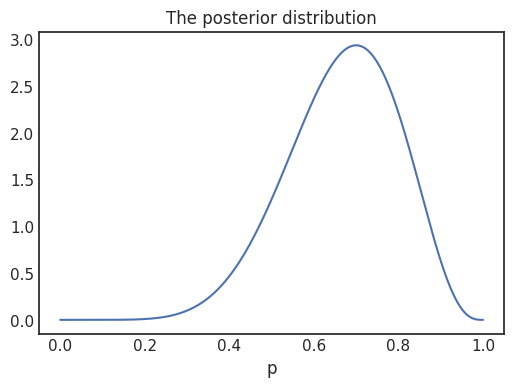### Maximum likelihood estimation

Even though we have a probability distribution, it is generally useful to provide a concrete number as our estimate. In this case, we would like to have a parameter estimate for the probability of our coin turning up heads. Although the Bayesian route was easy for coin tossing, it can be impossible to calculate analytically. One may ask the question: given our observed outcome, which parameter is the most likely? If you think about it, this is described by the likelihood function

$P(E | p) \propto p^7 (1 - p)^3$

where $E$ described the event $\text{HHTHTHHHHT}$. We calculated this by multiplying the probabilities of events we observed together.

In general, if we have the observations

$\vec{Y} = (Y_1, \dots, Y_n)$

with results

$\vec{y} = (y_1, \dots, y_n)$

written in vector form for notational convenience, the likelihood function is defined by

$P_{\vec{Y}}(\vec{y} | \theta) = \prod_{i=1}^{n} P_{Y_i}(y_i | \theta)$

which is a function of the variable $\textstyle \theta$. $\textstyle \theta$ denotes all parameters of our probability distribution. Thus it can be a scalar or a vector variable even. In our repeated coin-tossing example, the $\textstyle Y$-s denote the $\textstyle i$-th coin toss experiment, and $\textstyle y$ denotes the outcome. Here, $\textstyle y$ is one for heads and zero for tails. Also, keep in mind that in general, $\textstyle Y$ can be discrete or continuous.

Intuitively (a phrase we use quite a lot), the particular $\textstyle \theta$ value where the likelihood function assumes its maximum would be a reasonable choice for our parameter estimation. This method is called the maximum likelihood estimation or MLE in short. In mathematical terms,

$\widehat{\theta}_{\text{MLE}} = \arg \max_{\theta} P_{\vec{Y}}(\vec{y} | \theta).$

In the concrete example above, with seven heads and three tails, this is 0.7. Although MLE is not as desirable as the complete Bayesian treatment, it is often reasonable. Note that when the prior distribution is uniform, MLE is equivalent to estimating the parameter by maximizing the posterior distribution

$P(\theta | \vec{Y} = \vec{y}).$

This latter is called the maximum a posteriori estimation or MAP in short.

We are ready to revisit the original regression example with all of these mathematical tools under our belt!

## Regression revisited

To recap, we have the observations

${(x_1, y_1), \dots, (x_n, y_n)}$

and we would like to predict the $\textstyle y$-s from the $\textstyle x$-es. Previously, we were looking for a function $f(x) = ax + b$ such that

$\hat{y}_i = f(x_i)$

is reasonably close to the ground truth.

This time, let's look from a probabilistic viewpoint! Now we have data points

$\vec{x} = (x_1, \dots, x_n)$

coming from the distributions

$\vec{X} = (X_1, \dots, X_n)$

For each data point, we have ground truth observations

$\vec{y} = (y_1, \dots, y_n)$

coming from the distributions

$\vec{Y} = (Y_1, \dots, Y_n)$

It is reasonable to assume that all $\textstyle Y$-s can be modeled as a normal distribution $\mathcal{N}\big(\mu(x), \sigma(x)^2\big)$. For simplicity, we can assume that the variance is constant and that $\mu(x) = ax + b$ is a linear function. That is, we are looking to fit a model

$P_Y(y | X = x, q, b) = \mathcal{N}(y | ax + b, \sigma^2).$

Given all of our observations, we can write the likelihood function as

\begin{align*} P_{\vec{Y}}(\vec{y} | \vec{X} = \vec{x}, a, b) &= \prod_{i=1}^{n} P_{Y_i} (y_i | x_i, a, b) \\ &= \prod_{i=1}^{n} \mathcal{N}(y_i | a x_i + b, \sigma^2) \\ &= \prod_{i=1}^{n} \frac{1}{\sigma \sqrt{2 \pi}} e^{- \frac{(y_i - ax_i - b)^2}{(2 \sigma^2)}} \end{align*}

We want to maximize this function. It might seem complicated, but a standard mathematical trick is that maximizing a function is the same as maximizing its logarithm. (As long the logarithm is appropriately defined, like here.) So, we have

\begin{align*} \log P_{\vec{Y}}(\vec{y} | \vec{X} = \vec{x}, a, b) &= \sum_{i=1}^{n} \bigg[ \log \frac{1}{\sigma \sqrt{2 \pi}} - \frac{1}{2\sigma^2} (y_i - ax_i - b)^2 \bigg] \\ &= n \log \frac{1}{\sigma \sqrt{2 \pi}} - \frac{1}{2\sigma^2} \sum_{i=1}^{n} (y_i - ax_i - b)^2 \end{align*}

We see the light at the end of the tunnel here. The first term can be omitted (since it is a constant) while minimizing a function is the same as maximizing its negative. Thus,

$\arg \max_{a, b} P_{\vec{Y}}(\vec{y} | \vec{X} = \vec{x}, a, b) = \arg \min_{a, b} \sum_{i=1}^{n} (y_i - ax_i - b)^2$

It is no accident if it seems familiar: the right-hand side is the mean square error! This says that the maximum likelihood estimate for fitting a model in the form of

$\mathcal{N}(y | ax + b, \sigma^2)$

is the general case of the linear regression we did earlier. However, there is one major difference: the probabilistic model explains much more than a simple linear function. It is only the tip of the iceberg, and there are many ways to generalize this simple probabilistic model to obtain a more general fit for our data. One obvious method is to account for $\textstyle \sigma$ as a parameter and drop the constant assumption.

## Classification

Before we finish, we will take a detailed look at classification, another major class of problems in machine learning. Here, we have a slightly different kind of problem. Our training data is again

${(x_i, y_i)}_{i=1}^{n}$

where this time, the $\textstyle y$-s are labels instead of real numbers. Thus, a $\textstyle Y$ is a discrete probability distribution. Our previous linear regression model is unable to capture this problem properly. In addition, although mean square error can be used when the labels are encoded with integers, it doesn't really make sense.

For illustrative purposes, let's consider a simple binary classification problem in one dimension with two classes encoded with 0 and 1.If you are familiar with these types of problems, you probably know that the usual solution is to fit a function of the form

$f(x) = \sigma(ax + b),$

where

$\sigma(t) = \frac{1}{1 + e^{-t}}$

is the well-known Sigmoid function. This model is called logistic regression. Heuristically, the linear function is fitted such that it assumes positive values for $\textstyle x$-es belonging to the first class and negative values for the opposite class. Then, the Sigmoid converts these real numbers into probabilities. The higher $ax + b$ is, the closer its Sigmoid value is to 1, and similarly, the lower $ax + b$ is, the closer its Sigmoid value is to 0. Thus, $f(x)$ effectively models the probability that $x$ belongs to class 1.

To fit this model, the so-called cross-entropy loss is minimized, which is defined by

$\text{CE}(a, b) = -\sum_{i=1}^{n} \big[ y_i \log f(x_i) + (1 - y_i) \log (1 - f(x_i)) \big].$

What does this loss function mean? In the regression example at the very beginning, the mean square error was intuitively clear. This is not the case with cross-entropy. Have you ever thought about why cross-entropy loss is defined this way? By looking at this formula only, it is almost impossible to figure out.

If we look at the classification problem from the perspective we have gained in the previous sections, we can solve this binary classification problem by fitting a Bernoulli distribution to each $\textstyle x$. That is, we model our data with

$P_Y(y | X = x, a, b) = \text{Bernoulli}\big(\sigma(ax + b)\big).$

For the likelihood function, we have

\begin{align*} P_{\vec{Y}}(\vec{y} | \vec{X} = \vec{x}, a, b) &= \prod_{i=1}^{n} P_{Y_i}(y_i | x_i, a, b) \\ &= \prod_{i=1}^{n} \text{Bernoulli}\big( f(x_i) \big) \\ &= \prod_{i=1}^{n} f(x_i)^{y_i} \big(1 - f(x_i) \big)^{1 - y_i} \\ &= \prod_{i=1}^{n} \sigma(ax_i + b)^{y_i} \big( 1 - \sigma(ax_i + b) \big)^{1 - y_i}. \end{align*}

After taking logarithms like before, we obtain

$P_{\vec{Y}}(\vec{y} | \vec{X} = \vec{x}, a, b) = \sum_{i=1}^{n} \bigg[ y_i \log f(x_i) + (1 - y_i) \log \big( 1 - f(x_i) \big) \bigg],$

which is the negative of cross-entropy loss! All of a sudden, this mysterious formula filled with logarithms has a clear explanation. Minimizing the cross-entropy loss is simply modeling our data with Bernoulli distributions and taking the maximum likelihood estimate.

## Summary

When working with machine learning algorithms for problems such as classification and regression, we usually formulate questions in our spoken language like "What will be the price of this stock tomorrow?", "Is this a negative or positive comment?" and so on. I aimed to show that many of these algorithms are doing much more: they provide a deep statistical understanding of the underlying problem. Instead of simply estimating the stock price tomorrow, they can provide much more information than a simple prediction. We have seen that the fundamental objects of machine learning such as linear regression, binary classification with logistic regression, mean square error, and cross-entropy loss are all arising from very natural ideas in a statistical setting.

This is just the tip of the iceberg. Although we have seen only the most basic models, even the most advanced deep neural networks are built on these foundations. If you have understood these fundamentals, you are now one big step closer to master machine learning.